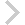FICO Xpress Optimization Examples Repository
 FICO Optimization Community FICO Xpress Optimization HomeUsing the model return value for error handling

Description
The default return value of a Mosel model at the end of its execution is 0. Other values may be set with the procedure exit as shown in the file exitvalue.mos. The model return values can be used by the calling application (exitvalue.c, runexitvalue.mos) for the implementation of error handling routines.

Source Files
By clicking on a file name, a preview is opened at the bottom of this page.

exitvalue.mos

(!*******************************************************
Mosel Example Problems
======================

file exitvalue.mos

Example of the use of exit'.

(c) 2008 Fair Isaac Corporation
author: S. Heipcke, 2002
*******************************************************!)

model "Using exit values"
uses "mmxprs"

declarations
R=1..10
A,B: array(R) of real
x: array(R) of mpvar
Ctr,obj: linctr
end-declarations

A:: [2, 4, 6, 8, 10, 9, 7, 5, 3, 1]
B:: [9, 7, 5, 3, 1, 2, 4, 6, 8, 10]

forall(r in R) x(r) is_binary
Ctr:=sum(r in R) B(r)*x(r) <= 100
Obj:=sum(r in R) A(r)*x(r)

! Solve the LP and test whether there is a solution
maximize(XPRS_LIN, Obj)
if(getprobstat=XPRS_INF) then
exit(1)
end-if

! Solve the MIP and test whether there is an integer solution
maximize(Obj)
stat:=getparam("XPRS_mipstatus")
if(stat<>XPRS_MIP_SOLUTION and stat<>XPRS_MIP_OPTIMAL) then
exit(2)
else
writeln("Solution: ", getobjval)
end-if

end-model

`